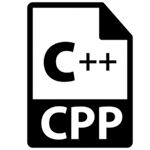# C++指针

C++语言中的指针是一个变量，它也称为定位符或指示符，它是指向一个值的地址。1. 指针减少代码并提高性能，它用于检索字符串，树等，并与数组，结构和函数一起使用。
2. 我们可以使用指针从函数返回多个值。
3. 它能够访问计算机内存中的任何内存位置。

1. 动态内存分配
在c语言中，可以使用malloc()和calloc()函数动态分配内存，其中使用的就是指针。

2. 数组，函数和结构体
C语言中的指针被广泛用于数组，函数和结构体中。 它减少了代码并提高了性能。

& 地址运算符 获取变量的地址。
* 间接运算符 访问地址的值。

## 声明指针

C++语言中的指针可以使用*(星号符号)声明。

```int ∗   a; //pointer to int
char ∗  c; //pointer to char```

## 指针示例

```#include <iostream>
using namespace std;
int main()
{
int number=30;
int ∗   p;
p=&number;//stores the address of number variable
cout<<"Value of p variable is:"<<*p<<endl;
return 0;
}```

```Address of number variable is:0x7ffccc8724c4
Value of p variable is:30```

```#include <iostream>
using namespace std;
int main()
{
int a=20,b=10,∗p1=&a,∗p2=&b;
cout<<"Before swap: ∗p1="<<∗p1<<" ∗p2="<<∗p2<<endl;
∗p1=∗p1+∗p2;
∗p2=∗p1-∗p2;
∗p1=∗p1-∗p2;
cout<<"After swap: ∗p1="<<∗p1<<" ∗p2="<<∗p2<<endl;
return 0;
}```

```Address of number variable is:0x7ffccc8724c4